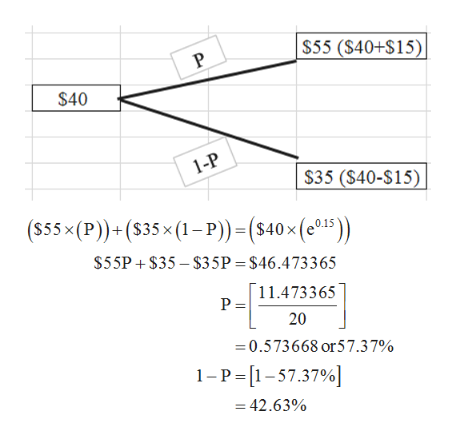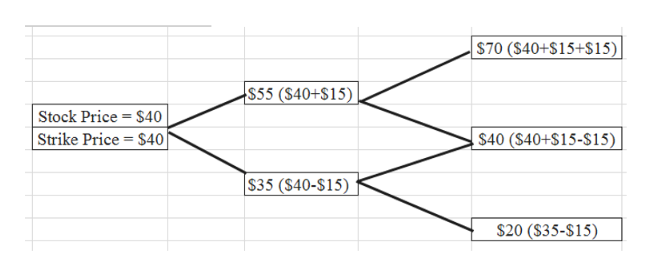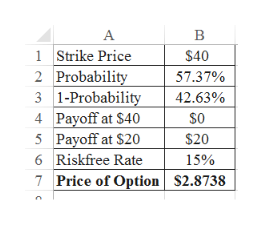# The current price of XL Corporation stock is \$40.  In each of the next two years, this stock price can either go up by \$15.00 or go down by \$15.00.  XL stock pays no dividends.  The one-year risk-free interest rate is 15% and will remain constant. Using the binomial pricing model, calculate the price of a two-year American straddle option on XL stock with a strike price of \$40.

Question
6 views

The current price of XL Corporation stock is \$40.  In each of the next two years, this stock price can either go up by \$15.00 or go down by \$15.00.  XL stock pays no dividends.  The one-year risk-free interest rate is 15% and will remain constant.

Using the binomial pricing model, calculate the price of a two-year American straddle option on XL stock with a strike price of \$40.

check_circle

Step 1

Calculate the probabilities:

p is 57.37% and 1-p is 42.63%.help_outlineImage TranscriptioncloseS55 (\$40+S15) P \$40 1-P \$35 (\$40-S15) (555x(P)+(S35x (1-P) =(s40x (e15 \$55P \$35-\$35P = \$46.4733 65 11.473365 P = 20 =0.573668 or57.3 7% 1-P [1-57.37% =42.63% fullscreen
Step 2

Create a binomial model:help_outlineImage Transcriptionclose\$70 (\$40+\$15+\$15) \$55 (\$40+\$15) Stock Price \$40 Strike Price = \$40 \$40 (\$40+\$15-\$15) \$35 (\$40-\$15) \$20 (\$35-\$15) fullscreen
Step 3

Calculate the Price of the Option:

Note that 2.71828 denotes the numerical value of exponential (e).

...help_outlineImage TranscriptioncloseB 1Strike Price 2 Probability 3 1-Probability 4 Payoff at \$40 5 Payoff at \$20 6 Riskfree Rate 7 Price of Option \$40 57.37% 42.63% \$0 \$20 15% \$2.8738 fullscreen

### Want to see the full answer?

See Solution

#### Want to see this answer and more?

Solutions are written by subject experts who are available 24/7. Questions are typically answered within 1 hour.*

See Solution
*Response times may vary by subject and question.
Tagged in